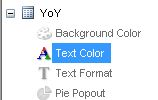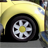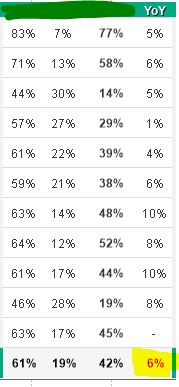# QlikView App Dev

Discussion Board for collaboration related to QlikView App Development.

Announcements
Our next Qlik Insider session will cover new key capabilities. Join us August 11th REGISTER TODAY
cancel
Showing results for
Did you mean:Contributor II

## Text Color Expression not working in totals of Straight Table

Hi everyone,

my first post/question here...

I have an issue with totals in a straight table. In the text color expression within my 'YoY' expression,I have calculated colors based on threshold values (positive = green, 0 = black, negative = red) , expression here:

 =If((\$(vCSATAMS) - \$(vDSATAMS))-(\$(vCSATL1AMS) - \$(vDSATL1AMS)) <0.005 and (\$(vCSATAMS) - \$(vDSATAMS))-(\$(vCSATL1AMS) - \$(vDSATL1AMS)) > -0.005, Black(), If((\$(vCSATAMS) - \$(vDSATAMS))-(\$(vCSATL1AMS) - \$(vDSATL1AMS)) >0.005, Green(), LightRed()))

Until here, everything works fine, but the totals. They are not following the text color expression and text color of total is always red, no matter if it's a positive or zero number. I have "average" in Total Mode, because with Expression Total it just doesn't show any totals.Any suggestion here?

Thanks

1 Solution

Accepted SolutionsPartner

Hi,

Try using Visual Cues Tab in Straight Table (ref. image attach)

OR

Custom Format Cell (ref. image attach)

9 RepliesPartner

Hi,

Try using Visual Cues Tab in Straight Table (ref. image attach)

OR

Custom Format Cell (ref. image attach)Specialist III

Hi,

If you want your totals to change, then you need to use Dimensionality() function. Where the Dimensionality() = 0 is the totals row.

Hope this helps.Specialist

I would follow what Sinan has recommended, you need to use Dimensionality() function to get the color right for TotalContributor II
Author

Hi @Sinan, thanks for the advice,

I'm not sure about how to use Dimensionality(), I've tried this but it's doing the same...

 =If(Dimensionality()<>0,If((\$(vCSATAMS) - \$(vDSATAMS))-(\$(vCSATL1AMS) - \$(vDSATL1AMS)) <0.005 and (\$(vCSATAMS) - \$(vDSATAMS))-(\$(vCSATL1AMS) - \$(vDSATL1AMS)) > -0.005, Black(), If((\$(vCSATAMS) - \$(vDSATAMS))-(\$(vCSATL1AMS) - \$(vDSATL1AMS)) >0.005, Green(), LightRed())), If(Dimensionality()=0,If((\$(vCSATAMS) - \$(vDSATAMS))-(\$(vCSATL1AMS) - \$(vDSATL1AMS)) <0.005 and (\$(vCSATAMS) - \$(vDSATAMS))-(\$(vCSATL1AMS) - \$(vDSATL1AMS)) > -0.005, Black(), If((\$(vCSATAMS) - \$(vDSATAMS))-(\$(vCSATL1AMS) - \$(vDSATL1AMS)) >0.005, Green(), LightRed()))))

Please, correct me if I'm wrong, thanks.Specialist III

Try the below:

=If((\$(vCSATAMS) - \$(vDSATAMS))-(\$(vCSATL1AMS) - \$(vDSATL1AMS)) <0.005 and

(\$(vCSATAMS) - \$(vDSATAMS))-(\$(vCSATL1AMS) - \$(vDSATL1AMS)) > -0.005, Black(),

If((\$(vCSATAMS) - \$(vDSATAMS))-(\$(vCSATL1AMS) - \$(vDSATL1AMS)) >0.005 And Dimensionality()>=0, Green(), LightRed()))

Hope this works.Contributor II
Author

Thanks Sinan, but still not working...

Actually, if I put only Dimensionality()=0, the color is only in totals, but still red.Any other suggestion? 😕Contributor II
Author

Visual cues did it!

I was trying to do it inside the expression, but this worked perfectly anyway.

Thanks Hiren!!Specialist III

Can you post the app? or just a small portion of it at least? Well, if you want to color change to be all the expressions including the total row, then you use Dimensionality() >= 0. If you only want the total row to change, then Dimensionality() = 0.

A sample app would be helpful at this point.

ThanksContributor II
Author

Thanks for the help Sinan,

I'd tried with both (>=0 and =0) and total remains red.

I did it with visual cues as Hiren recommended. But I will try with dimensionality too and will let you know how it's going.

Thanks for the helpCommunity Browser